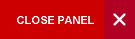#### Online Support

Level I (Minimum 30 credits)

1. ECON1000: Principles of Economics I
2. ECON1012: Principles of Economics II
3. ECON1005: Introductory Statistics
4. MATH1141: Intro. Linear Algebra & Analytic Geometry
5. MATH1142: Calculus I
6. MATH1151: Calculus II
7. MATH1152: Introduction to Formal Mathematics
8. FOUN1013: Critical Reading & Writing in Soc. Sciences or FOUN1019: Critical Reading and Writing in the Disciplines
9. FOUN1101: Caribbean Civilization
10. FOUN1201: Science, Medicine & Technology in Society

Level II & Level III (Minimum 66 credits)

11. ECON2000: Intermediate Microeconomics I
12. ECON2001: Intermediate Microeconomics II
13. ECON2002: Intermediate Macroeconomics I
14. ECON2003: Intermediate Macroeconomics II
15. MATH2401: Elements of Mathematical Analysis
16. MATH2403: Multivariable Calculus
17. MATH2404: Introduction to Probability Theory
18. MATH2410: A First Course in Linear Algebra
19. MATH2411: Introduction to Abstract Algebra
20. MATH2420: Ordinary Differential Equations
21. ECON3049: Econometrics
22. MATH3155: Complex Variables
24. MATH3402: Metric Spaces and Topology
25-27. Plus 3 Level II/III Economics Electives
28-29. Plus 2 Level III Economics Electives
30-32. Plus 3 Mathematics Electives*

NOTE:

The following are considered as Mathematics Electives:
- MATH2407: Stochastic Modelling
- MATH2420: Ordinary Differential Equations
- MATH2421: Fourier Series & Integral Transforms
- MATH2430: Linear Optimization
- MATH2431: Non-Linear Optimization
- MATH3401: Introduction to the Theory of Integration
- MATH3402: A Course on Metric Space & Topology
- MATH3403: Some Topics in Functional Analysis 6
- MATH3404: Introduction to Differential Geometry with Computer Software
- MATH3414: Selected Topics in Operations Research
- MATH3421: Partial Differential Equations
- MATH3422: Mathematical Modelling
- MATH3424: Numerical Methods
- STAT2001: Inferential Statistics
- STAT3001: Regression Analysis
- STAT3002: Time Series

II. Mathematics students who choose this double major should select courses from the MATHEMATICS AND ECONOMICS option offered by the Mathematics Department.
III. For students pursuing double Majors in Economics and Mathematics, where courses are anti-requisites, students should substitute the Economics courses with other Levels II/III Economics electives.

Top of Page